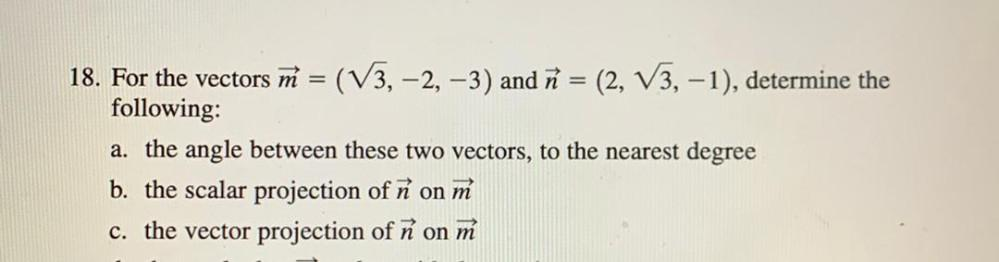Question:

# For the vectors M = (√3, -2, -3) and n = (2, √3, -1), determine the following: a). the angle between these two vectors, to the nearest degree b). the scalar projection of ñ on m c). the vector projectFor the vectors M = (√3, -2, -3) and n = (2, √3, -1), determine the following: a). the angle between these two vectors, to the nearest degree b). the scalar projection of ñ on m c). the vector projection of n on m